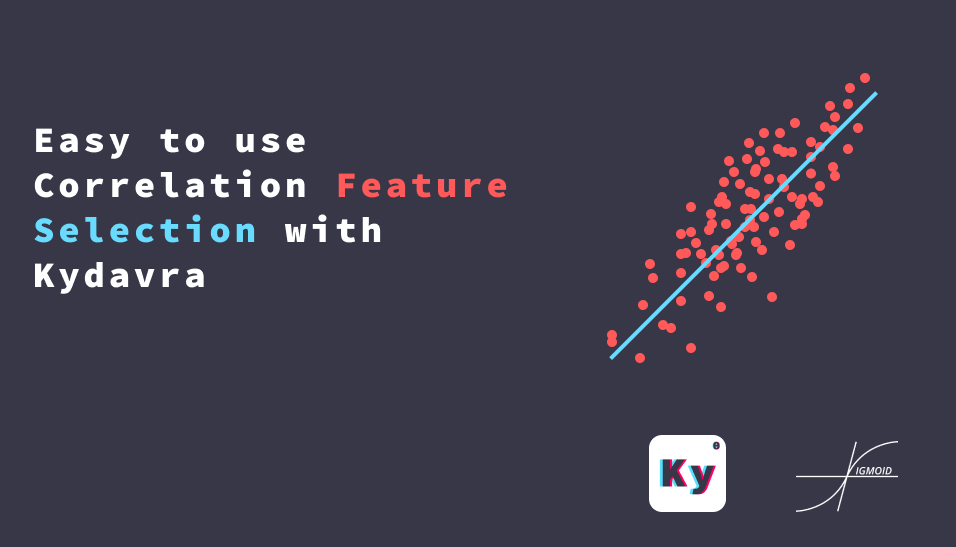Join thousands of AI enthusiasts and experts at the Learn AI Community.

## PublicationMachine Learning

# Easy to use Correlation Feature Selection with Kydavra

Last Updated on August 24, 2020 by Editorial Team

#### Machine Learning

Almost every person in data science or Machine Learning knows that one of the easiest ways to find relevant features for predicted value y is to find the features that are most correlated with y. However few (if not a mathematician) know that there are many types of correlation. In this article, I will shortly tell you about the 3 most popular types of Correlation and how you can easily apply them with Kydavra for feature selection.

Pearson correlation.

Pearson’s correlation coefficient in the covariance of two variables divided by the product of their standard deviations.Figure 1. The formula to calculate the Pearson correlation between 2 features.

It’s valued between -1 and 1, negative values meaning inverse relation and positive, the reverse case. Often we just take the absolute value. So if the absolute value is above 0.5 the series can have (yes can have) a relation. However, we also set a vertical limit, 0.7 or 0.8, because if values are too correlated then possibly one series is derived from another (like age in months from age in years) or simply can drive our model to overfitting.

Using Kydavra PearsonCorrelationSelector.

Firstly you should install kydavra, if you don’t have it installed.

`pip install kydavra`

Next, we should create an abject and apply it to the Hearth Disease UCI dataset.

`from kydavra import PearsonCorrelationSelector`
`selector = PearsonCorrelationSelector()`
`selected_cols = selector.select(df, ‘target’)`

Applying the default setting of the selector on the Hearth Disease UCI Dataset will give us an empty list. This is because no feature has a correlation with the target feature higher than 0.5. That’s why we highly recommend you play around with parameters of the selector:

• min_corr (float, between 0 and 1, default=0.5) the minimal value of the correlation coefficient to be selected as an important feature.
• max_corr (float, between 0 and 1, default=0.5) the minimal value of the correlation coefficient to be selected as an important feature.
• erase_corr (boolean, default=False) if set to True then the algorithm will erase columns that are correlated between keeping just on, if False then it will keep all columns.

The last feature was implemented because if you are building a model with 2 features that are highly correlated with each other, then you practically are giving the same information creating the problem of multilinearity. So changing the min_corr to 0.3 gives the next columns:

`['sex', 'cp', 'thalach', 'exang', 'oldpeak', 'slope', 'ca', 'thal']`

and the cross-validation score remains the same — 0.81. A good result.

Spearman Correlation.

When Pearson correlation is based on the assumption that data is normally distributed, Spearman rank coefficient doesn’t make this assumption. So the values are different. However, the Spearman rank coefficient is also ranged by -1, and 1. The mathematical details of how it is calculated are out of the scope of this article so, below are some articles that analyze it (and the next type of correlation in more detail).

So now let’s apply SpermanCorrelationSelector to our Dataset.

`from kydavra import SpermanCorrelationSelector`
`selector = SpermanCorrelationSelector()`
`selcted_cols = selector.select(df, ‘target’)`

Using default setting the selector also returns an empty list. But setting the min_corr to 0.3 gives the same column as PearsonCorrelation. The parameters are the same for all Correlation Selectors.

Kendall Rank Correlation.

Kendall Rank Correlation is also implemented in the Kydavra library. We let theory on articles that dive deeper into it. So to use Kendall Rank Correlation use the following template.

`from kydavra import KendallCorrelationSelector`
`selector = KendallCorrelationSelector()`
`selected_cols = selector.select(df, ‘target’)`

Testing its performance we also let on you. Below are some articles that dive into more depth the Correlation metrics.

If you used or tried Kyadavra we highly invite you to fill this form and share your experience.

Made with ❤ by Sigmoid.

#### ResourcesEasy to use Correlation Feature Selection with Kydavra was originally published in Towards AI — Multidisciplinary Science Journal on Medium, where people are continuing the conversation by highlighting and responding to this story.

Published via Towards AI